java泛型与反射总结android面试准备 同时被 2 个专栏收录26 篇文章 1 订阅

泛型基础

泛型的好处

//不使用泛型需要强制转换
List list = new ArrayList();
System.out.println(list.get(0));
System.out.println((String)list.get(1));% class java.lang.Integer cannot be cast to class java.lang.String
//使用泛型可避免强制转换
List<String> list = new ArrayList<String>();
String s = list.get(0);

泛型使用

public class ObjectTool<T> {
private T obj;
public T getObj() {
return obj;
}
public void setObj(T obj) {
this.obj = obj;
}
}

public class ObjectTool {
public <T> T show(T t) {
if (t != null){
return t;
}else{
return null;
}
}

public class Ttry {
public static void main(String[]args){
showTry showTry = new showTry();
System.out.println(showTry.show(20));
}
}
interface show<T>{
T show(T t);
}
class showTry implements show<Integer>{
public showTry(){

}
@Override
public Integer show(Integer num){
return  num;
}
}

类型变量的限定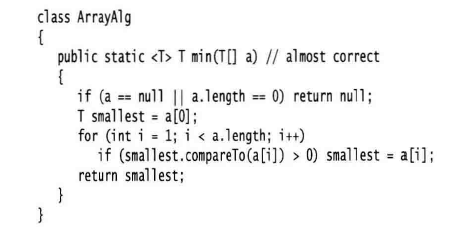public static<T extends Comparable> T min(T[]a)
T extends Comparable & Serializable

类型擦除

类型擦除概述

class Pair<T>{
private T first;
public Pair(T first) this.first = first;
public T getFirst(){return first;}
public void setFirst(T newvalue)first = newvalue;
}
class Pair{
private Object first;
public Pair(Object first) this.first = first;
public Object getFirst(){return first;}
public void setFirst(Object newvalue)first = newvalue;
}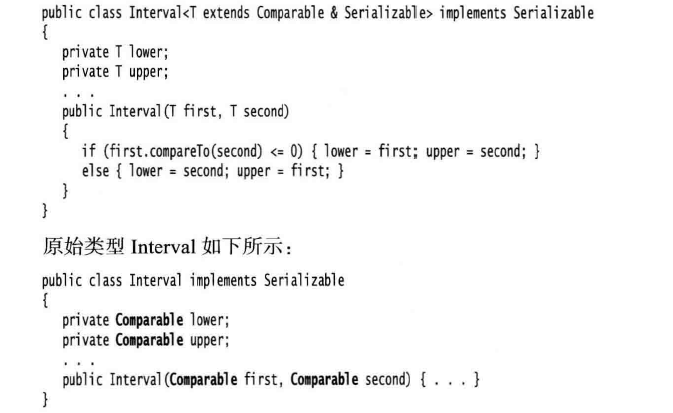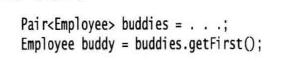public static <T extends Comparable> T min(T[]a)
public static Comparable min(Comparable a)

类型擦除带来的影响

1.不能使用基本类型作为类型变量,类型擦除之后List含有Object的域，而Object域不能存储int值。

List<int>list = new ArrayList<>();//error
List<Integer>list = new ArrayList<>();

2.运行时类型查询仅适用于原始类型

if (list instanceof ArrayList<Integer>)//error
if (list instanceof ArrayList<T>)//error
//其实查询的只是判断list是否是任意一个类型的ArrayList
if(list instanceof ArrayList<?>)//ok
ArrayList<String>()list1 = new ArrayList<>();
ArrayList<Integer>list2 = new ArrayList<>();
list1.getClass()==list2.getClass();//return true,都是ArrayList.class

3.不能创建参数化类型的数组
List和 List在 jvm 中等同于List，所有的类型信息都被擦除，程序也无法分辨一个数组中的元素类型具体是 List类型还是 List类型。

List<Integer>[] li2 = new ArrayList<Integer>;//error
List<Boolean> li3 = new ArrayList<Boolean>;//error
List<List<Integer>>lists = new ArrayList;//ok

4.不能实例化类型变量

public Pair(){first = new T();second = new T();}//error

5.不能抛出或捕获泛型类的实例

//不能创建、捕捉或抛出参数化类型的对象
class MathException<T> extends Exception {}    // error
class QueueFullException<T> extends Throwable {} // error
public static <T extends Exception, J> void execute(List<J> jobs) {
try {
} catch (T e) {   // error
}
}

6.不能重载参数类型为相同原始类型的方法

public class Example { // error
public void print(List<String> list) {}
public void print(List<Integer> list) {}
}

通配符类型

1.？extends Employee，而当前代码代表它的参数类型是Employee的子类，但一定不是其他的比如String。

Pair<? extends Employee>

public static void returnNum(MyChar<A> c)
public static void returnNum(MyChar<? extends A> c)
public class Ttry {
public static void main(String[]args){
//showTry showTry = new showTry();
//System.out.println(showTry.show(20));
A a = new A(10);
B b = new B(20,30);
MyChar<A> aMyChar = new MyChar<>(a);
MyChar<B> bMyChar = new MyChar<>(b);
returnNum(aMyChar);
returnNum(bMyChar);
}
public static void returnNum(MyChar<? extends A> c){
A a = c.getT();
System.out.println(a.getNum());
}
}
class  MyChar<T>{
private T first;
public MyChar(T t)
{
this.first = t;
}
public T getT(){
return first;
}
}
class  A{
private int num;
public A(int num)
{
this.num = num;
}
public int getNum(){
return num;
}
}
class B extends A{
private int size;
public B(int num){
super(num);
}
public B(int num,int size)
{
super(num);
this.size = size;
}
public  int getNum()
{
return  super.getNum();
}
}

2.？super Employee代表当前通配符限制为Employee的父类。

3.还可以使用无限定通配符：“？”

反射

java反射机制：在程序运行时，能知道任意一个类的所有属性和方法，对于任意一个对象都能调用它的任意一个方法和属性。这种动态的获取信息或动态的调用对象的方法称之为反射。

反射基础

TestClass testClass = new TestClass();
Class mClass = testClass.getClass();

TestClass testClass = new TestClass();
testClass.getName().newInstance();

使用反射获取类的信息

FatherClass.java

public class FatherClass {
public String mFatherName;
public int mFatherAge;

public void printFatherMsg(){}
}

SonClass.java

public class SonClass extends FatherClass{

private String mSonName;
protected int mSonAge;
public String mSonBirthday;

public void printSonMsg(){
System.out.println("Son Msg - name : "
+ mSonName + "; age : " + mSonAge);
}

private void setSonName(String name){
mSonName = name;
}

private void setSonAge(int age){
mSonAge = age;
}

private int getSonAge(){
return mSonAge;
}

private String getSonName(){
return mSonName;
}
}

获取类的所有变量信息

/**
* 通过反射获取类的所有变量
*/
private static void printFields(){
//1.获取并输出类的名称
Class mClass = SonClass.class;
System.out.println("类的名称：" + mClass.getName());

//2.1 获取所有 public 访问权限的变量
// 包括本类声明的和从父类继承的
Field[] fields = mClass.getFields();

//2.2 获取所有本类声明的变量（不问访问权限）
//Field[] fields = mClass.getDeclaredFields();

//3. 遍历变量并输出变量信息
for (Field field :
fields) {
//获取访问权限并输出
int modifiers = field.getModifiers();
System.out.print(Modifier.toString(modifiers) + " ");
//输出变量的类型及变量名
System.out.println(field.getType().getName()
+ " " + field.getName());
}
}

public java.lang.String mSonBirthday
public java.lang.String mFatherName
public int mFatherAge

private java.lang.String mSonName
protected int mSonAge
public java.lang.String mSonBirthday

获取类的所有方法信息

/**
* 通过反射获取类的所有方法
*/
private static void printMethods(){
//1.获取并输出类的名称
Class mClass = SonClass.class;
System.out.println("类的名称：" + mClass.getName());

//2.1 获取所有 public 访问权限的方法
//包括自己声明和从父类继承的
Method[] mMethods = mClass.getMethods();

//2.2 获取所有本类的的方法（不问访问权限）
//Method[] mMethods = mClass.getDeclaredMethods();

//3.遍历所有方法
for (Method method :
mMethods) {
//获取并输出方法的访问权限（Modifiers：修饰符）
int modifiers = method.getModifiers();
System.out.print(Modifier.toString(modifiers) + " ");
//获取并输出方法的返回值类型
Class returnType = method.getReturnType();
System.out.print(returnType.getName() + " "
+ method.getName() + "( ");
//获取并输出方法的所有参数
Parameter[] parameters = method.getParameters();
for (Parameter parameter:
parameters) {
System.out.print(parameter.getType().getName()
+ " " + parameter.getName() + ",");
}
//获取并输出方法抛出的异常
Class[] exceptionTypes = method.getExceptionTypes();
if (exceptionTypes.length == 0){
System.out.println(" )");
}
else {
for (Class c : exceptionTypes) {
System.out.println(" ) throws "
+ c.getName());
}
}
}
}

**获取 SonClass 类所有 public 访问权限的方法，包括从父类继承的。**打印信息中，printSonMsg() 方法来自 SonClass 类， printFatherMsg() 来自 FatherClass 类，其余方法来自 Object 类。

public void printSonMsg(  )
public void printFatherMsg(  )
public final void wait(  ) throws java.lang.InterruptedException
public final void wait( long arg0,int arg1, ) throws java.lang.InterruptedException
public final native void wait( long arg0, ) throws java.lang.InterruptedException
public boolean equals( java.lang.Object arg0, )
public java.lang.String toString(  )
public native int hashCode(  )
public final native java.lang.Class getClass(  )
public final native void notify(  )
public final native void notifyAll(  )

访问以及操作类的私有变量及方法（重要）

public class TestClass {

private String MSG = "Original";

private void privateMethod(String head , int tail){
}

public String getMsg(){
return MSG;
}
}

访问私有方法

/**
* 访问对象的私有方法
* 为简洁代码，在方法上抛出总的异常，实际开发别这样
*/
private static void getPrivateMethod() throws Exception{
//1. 获取 Class 类实例
TestClass testClass = new TestClass();
Class mClass = testClass.getClass();

//2. 获取私有方法
//第一个参数为要获取的私有方法的名称
//第二个为要获取方法的参数的类型，参数为 Class...，没有参数就是null
//方法参数也可这么写 ：new Class[]{String.class , int.class}
Method privateMethod =
mClass.getDeclaredMethod("privateMethod", String.class, int.class);

//3. 开始操作方法
if (privateMethod != null) {
//获取私有方法的访问权
//只是获取访问权，并不是修改实际权限
privateMethod.setAccessible(true);

//使用 invoke 反射调用私有方法
//privateMethod 是获取到的私有方法
//testClass 要操作的对象
//后面两个参数传实参
privateMethod.invoke(testClass, "Java Reflect ", 666);
}
}

访问（更改）私有变量

/**
* 修改对象私有变量的值
* 为简洁代码，在方法上抛出总的异常
*/
private static void modifyPrivateFiled() throws Exception {
//1. 获取 Class 类实例
TestClass testClass = new TestClass();
Class mClass = testClass.getClass();

//2. 获取私有变量
Field privateField = mClass.getDeclaredField("MSG");

//3. 操作私有变量
if (privateField != null) {
//获取私有变量的访问权
privateField.setAccessible(true);

//修改私有变量，并输出以测试
System.out.println("Before Modify：MSG = " + testClass.getMsg());

//调用 set(object , value) 修改变量的值
//privateField 是获取到的私有变量
//testClass 要操作的对象
//"Modified" 为要修改成的值
privateField.set(testClass, "Modified");
System.out.println("After Modify：MSG = " + testClass.getMsg());
}
}

Before Modify：MSG = Original
After Modify：MSG = Modified

修改私有常量

常规情况

//注意是 String  类型的值
private final String FINAL_VALUE = "hello";

if(FINAL_VALUE.equals("world")){
//do something
}

private final String FINAL_VALUE = "hello";
//替换为"hello"
if("hello".equals("world")){
//do something
}

//String 会被 JVM 优化
private final String FINAL_VALUE = "FINAL";

public String getFinalValue(){
//剧透，会被优化为: return "FINAL" ,拭目以待吧
return FINAL_VALUE;
}

/**
* 修改对象私有常量的值
* 为简洁代码，在方法上抛出总的异常，实际开发别这样
*/
private static void modifyFinalFiled() throws Exception {
//1. 获取 Class 类实例
TestClass testClass = new TestClass();
Class mClass = testClass.getClass();

//2. 获取私有常量
Field finalField = mClass.getDeclaredField("FINAL_VALUE");

//3. 修改常量的值
if (finalField != null) {

//获取私有常量的访问权
finalField.setAccessible(true);

//调用 finalField 的 getter 方法
//输出 FINAL_VALUE 修改前的值
System.out.println("Before Modify：FINAL_VALUE = "
+ finalField.get(testClass));

//修改私有常量
finalField.set(testClass, "Modified");

//调用 finalField 的 getter 方法
//输出 FINAL_VALUE 修改后的值
System.out.println("After Modify：FINAL_VALUE = "
+ finalField.get(testClass));

//使用对象调用类的 getter 方法
//获取值并输出
System.out.println("Actually ：FINAL_VALUE = "
+ testClass.getFinalValue());
}
}

Before Modify：FINAL_VALUE = FINAL
After Modify：FINAL_VALUE = Modified
Actually ：FINAL_VALUE = FINAL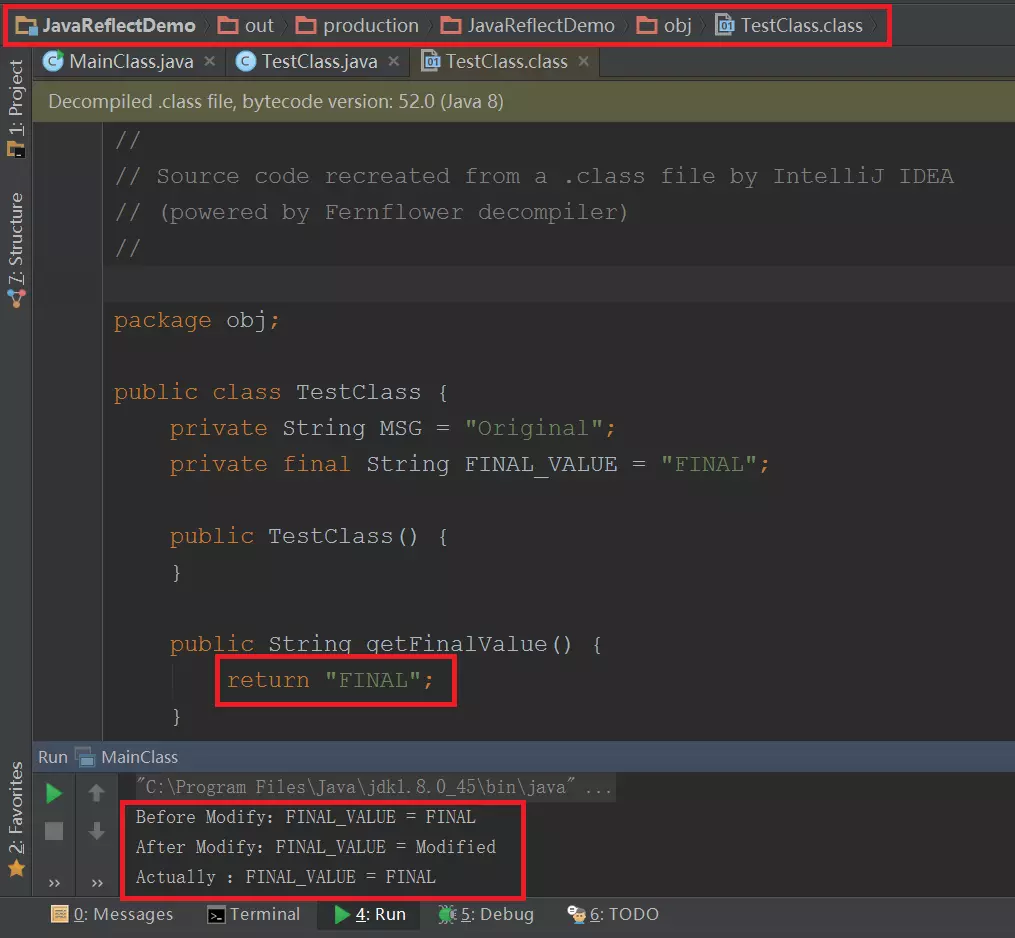非常规情况

java允许我们不在声明时赋值，可以在构造函数再赋值。

public class TestClass {

//......
private final String FINAL_VALUE;

//构造函数内为常量赋值
public TestClass(){
this.FINAL_VALUE = "FINAL";
}
//......
}

Before Modify：FINAL_VALUE = FINAL
After Modify：FINAL_VALUE = Modified
Actually ：FINAL_VALUE = Modified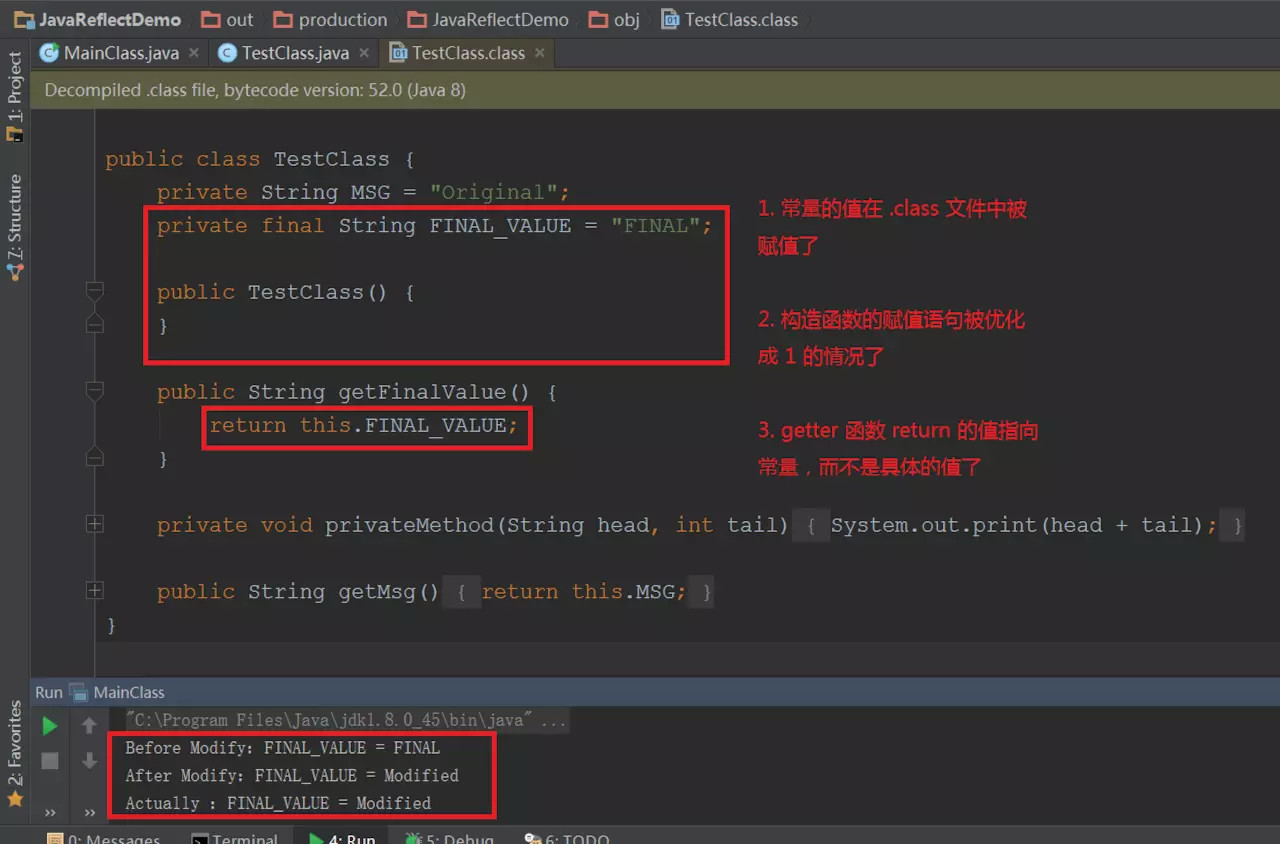泛型与反射的结合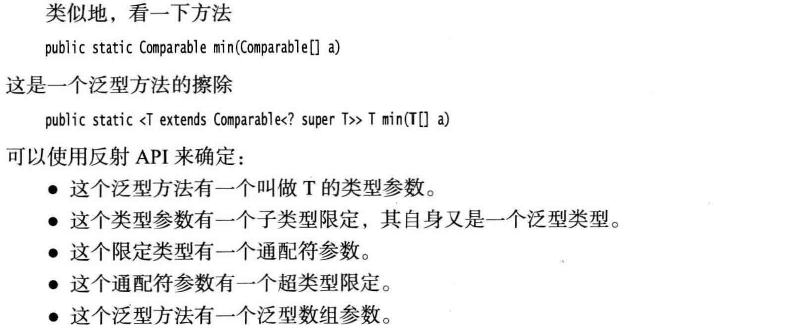使用反射编写泛型数组

java.lang.reflect包中的Array允许动态的创建数组。例如CopyOf方法，可以将数组扩充至想要的长度。

int[]num = new int[]{5,4,3,2};
num = Arrays.copyOf(num,10);
System.out.println(num.length);%10

Object[] xin = new Object[length];
System.arraycopy(num,0,xin,0,length);
return xin;
}

public static Object goodCopyOf(Object num,int length){
Class c1 = num.getClass();
//获取数组的元素类型
Class commpentType = c1.getComponentType();
int oldlength = Array.getLength(num);
Object newArray = Array.newInstance(commpentType,length);
System.arraycopy(num,0,newArray,0,Math.min(length,oldlength));
return newArray;
}

泛型与反射的总结（复习必看）

1.泛型总结

2.泛型类：class A<T>

3.类型变量T可以有限定，比如当我们想调用类型变量的compare方法，就要求类型变量T所对应的那些类都实现了Comparable接口，虽然是接口，我们也使用extends关键词。<T extends Comparable>

4.类型擦除虚拟机中没有泛型类型对象，会对其进行类型擦除。如果只是类型变量T，则会将其擦除为Object，如果<T extends Comparable>，就会擦除为Comparabale。

//例子1
class Pair<T>{
private T first;
public Pair(T first) this.first = first;
public T getFirst(){return first;}
public void setFirst(T newvalue)first = newvalue;
}
class Pair{
private Object first;
public Pair(Object first) this.first = first;
public Object getFirst(){return first;}
public void setFirst(Object newvalue)first = newvalue;
}
//例子2
public static <T extends Comparable> T min(T[]a)
public static Comparable min(Comparable a)

5.类型擦除带来的影响
1）不能使用基本类型作为类型变量，而要使用其包装类。

List<int>list = new ArrayList<>();//error
List<Integer>list = new ArrayList<>();

2）不能使用instanceOfgetclass运行时类型查询仅适用于原始类型。虚拟机中的对象有一个特定的非泛型类型，因此所有的类查询都只会产生原始类型。

if (list instanceof ArrayList<Integer>)//error
ArrayList<String>()list1 = new ArrayList<>();
ArrayList<Integer>list2 = new ArrayList<>();
list1.getClass()==list2.getClass();//return true,都是ArrayList.class

3）不能创建参数化类型数组

List<Integer>[] li2 = new ArrayList<Integer>;//error
List<Boolean> li3 = new ArrayList<Boolean>;//error
List<List<Integer>>lists = new ArrayList;//ok

4）不能实例化类型变量

public Pair(){first = new T();second = new T();}//error

5）不能抛出或捕获泛型类的实例

//不能创建、捕捉或抛出参数化类型的对象
class MathException<T> extends Exception {}    // error
class QueueFullException<T> extends Throwable {} // error
public static <T extends Exception, J> void execute(List<J> jobs) {
try {
} catch (T e) {   // error
}
}

6）不能重载参数类型为相同原始类型的方法

public class Example { // error
public void print(List<String> list) {}
public void print(List<Integer> list) {}
}

6.通配符，可以使用通配符来让约束类型变量，<？ extends A>,则要求传入的数据类型必须是A的子类，
？ super A,则要求传入的数据类型必须是A的父类。

反射总结

1.反射的作用：可以通过反射在运行时动态的获取任意类的任意成员变量及任意方法，以及动态的进行访问任意成员变量及任意方法（包括private方法与private对象）当类中存在私有常量，反射也可以进行更改，但如果私有常量在声明时赋值，则无法对其相关的方法产生影响。（因为虚拟机会在编译时将.java文件得到.class文件时，会将静态常量的引用代码都直接更改为静态常量的具体数值）。

2.获取类的变量信息与获取类的方法信息类似。创建Class对象，并分别通过getFields()getMethods()方法可以获取到类的变量与方法。注意这里获取到的只有当前类及其父类的public方法与public的成员变量。

3.反射可以访问私有方法和私有对象。都需要通过privateMethod.setAccessible(true)获取访问权，对应私有方法就使用privateMethod.invoke（对象，形参）方法来调用privateMethod；对应私有对象就使用`privateField.set(对象, 要修改成的值);

4.修改私有常量的方法需要在构造函数中对私有常量赋值才可以，否则则无法对引用私有常量的方法起到作用。

5.由于泛型会被类型擦除，使用反射的type接口，可以来确定信息。同时，使用反射可以构建泛型数组。因为通过Class commpentType = c1.getComponentType();可以获取到数组的元素类型，进而创建相应的数组Object newArray =newInstance(commpentType,length)。只暂时让数组为Object，之后再转换类型num = （int）Arrays.goodcopyOf(num,10);

03-27228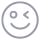插入表情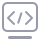代码片
08-093600
02-12266
05-052553
06-121232
11-20434
09-13
07-033102
03-2156
07-07296
10-20220
05-15454
07-05174
02-20195
02-12257
10-211万+
07-2856
05-10182点击重新获取扫码支付余额充值